This section describes the methodology and the fitting procedure behind generalized additive models.

Let Y be a response random variable and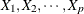be a set of predictor variables. A regression procedure can be viewed as a method for estimating the expected value of Y given the values of. The standard linear regression model assumes a linear form for the dependency of Y on X: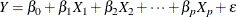where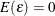and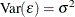. Given a sample, estimates of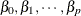are usually obtained by the least squares method.

The additive model generalizes the linear model by modeling the dependency as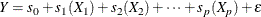where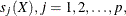are smooth functions,and.

In order to be estimable, the smooth functions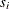have to satisfy standardized conditions such as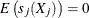. These functions are not given a parametric form but instead are estimated in a nonparametric fashion.

While traditional linear models and additive models can be used in most statistical data analysis, there are types of problems for which they are not appropriate. For example, the normal distribution might not be adequate for modeling discrete responses such as counts or bounded responses such as proportions.

Generalized additive models address these difficulties, extending additive models to many other distributions besides just the normal. Thus, generalized additive models can be applied to a much wider range of data analysis problems.

Like generalized linear models, generalized additive models consist of a random component, an additive component, and a link function relating the two components. The response Y, the random component, is assumed to have exponential family density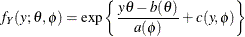whereis called the natural parameter and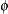is the scale parameter. The mean of the response variableis related to the set of covariatesby a link function g. The quantity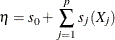defines the additive component, where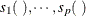are smooth functions, and the relationship betweenand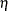is defined by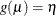. The most commonly used link function is the canonical link, for which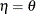.

Generalized additive models and generalized linear models can be applied in similar situations, but they serve different analytic purposes. Generalized linear models emphasize estimation and inference for the parameters of the model, while generalized additive models focus on exploring data nonparametrically. Generalized additive models are more suitable for exploring the data and visualizing the relationship between the dependent variable and the independent variables.